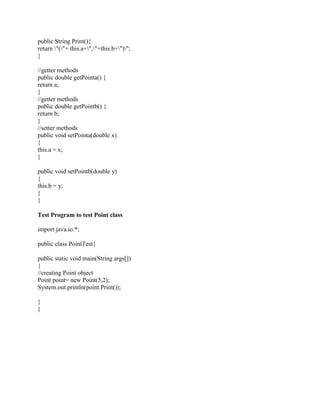Diese Präsentation wurde erfolgreich gemeldet.

# Write a class called point in JAVA for the 2-D environment- Select app.docx

Anzeige
Anzeige
Anzeige
Anzeige
Anzeige
Anzeige
Anzeige
Anzeige
Anzeige
Anzeige
Anzeige×

1 von 3 Anzeige

# Write a class called point in JAVA for the 2-D environment- Select app.docx

Write a class called point in JAVA
for the 2-D environment.
Select appropriate variable(s).
Write constructors.
Write access methods for the variables.
Write a Print method to display the variables when called.
Write a test program to test it.
-------------------------------------------------------------------------------
Write a class called square,
which inheres the above point class in JAVA
Select appropriate variable(s).
Write constructors.
Write access methods for the variables.
Override the inherited print method to display variable in square and point.
Write a test program to test it.
Solution
Point class is given below with proper comments
import java.io.*;
public class Point
{
private double a;
private double b;
public Point(double x, double y)
{
this.a=x;
this.b=y;
}
public String Print(){
return \"(\"+ this.a+\",\"+this.b+\")\";
}
//getter methods
public double getPointa() {
return a;
}
//getter methods
public double getPointb() {
return b;
}
//setter methods
public void setPointa(double x)
{
this.a = x;
}
public void setPointb(double y)
{
this.b = y;
}
}
Test Program to test Point class
import java.io.*;
public class PointTest{
public static void main(String args[])
{
//creating Point object
Point point= new Point(5,2);
System.out.println(point.Print());

}
}
.

Write a class called point in JAVA
for the 2-D environment.
Select appropriate variable(s).
Write constructors.
Write access methods for the variables.
Write a Print method to display the variables when called.
Write a test program to test it.
-------------------------------------------------------------------------------
Write a class called square,
which inheres the above point class in JAVA
Select appropriate variable(s).
Write constructors.
Write access methods for the variables.
Override the inherited print method to display variable in square and point.
Write a test program to test it.
Solution
Point class is given below with proper comments
import java.io.*;
public class Point
{
private double a;
private double b;
public Point(double x, double y)
{
this.a=x;
this.b=y;
}
public String Print(){
return \"(\"+ this.a+\",\"+this.b+\")\";
}
//getter methods
public double getPointa() {
return a;
}
//getter methods
public double getPointb() {
return b;
}
//setter methods
public void setPointa(double x)
{
this.a = x;
}
public void setPointb(double y)
{
this.b = y;
}
}
Test Program to test Point class
import java.io.*;
public class PointTest{
public static void main(String args[])
{
//creating Point object
Point point= new Point(5,2);
System.out.println(point.Print());

}
}
.

Anzeige
Anzeige

### Write a class called point in JAVA for the 2-D environment- Select app.docx

1. 1. Write a class called point in JAVA for the 2-D environment. Select appropriate variable(s). Write constructors. Write access methods for the variables. Write a Print method to display the variables when called. Write a test program to test it. ------------------------------------------------------------------------------- Write a class called square, which inheres the above point class in JAVA Select appropriate variable(s). Write constructors. Write access methods for the variables. Override the inherited print method to display variable in square and point. Write a test program to test it. Solution Point class is given below with proper comments import java.io.*; public class Point { private double a; private double b; public Point(double x, double y) { this.a=x; this.b=y; }
2. 2. public String Print(){ return "("+ this.a+","+this.b+")"; } //getter methods public double getPointa() { return a; } //getter methods public double getPointb() { return b; } //setter methods public void setPointa(double x) { this.a = x; } public void setPointb(double y) { this.b = y; } } Test Program to test Point class import java.io.*; public class PointTest{ public static void main(String args[]) { //creating Point object Point point= new Point(5,2); System.out.println(point.Print()); } }